## Creating a dynamic response of an API/Microservice

Hello Guys!

Today, I’m going to discuss a potential use case, where on many occasions, different teams need almost similar kinds of data through API. However, they are not identical. Creating a fresh API/Microservice after following-up with many processes will take significant time.

What if we can create an API in such a way so that we can get the response dynamically without needing to make another one. In this post, we’ll be demonstrating a similar approach.

I’ll be using open-source Covid-API, which will be useful for several posts starting from this one.

You will get plenty of useful data from here.

We’ve chosen the following one for our use case –

Let’s explore the sample data first.

```[
{
"date":20210207,
"state":"AK",
"positive":53279.0,
"probableCases":null,
"negative":null,
"pending":null,
"totalTestResultsSource":"totalTestsViral",
"totalTestResults":1536911.0,
"hospitalizedCurrently":44.0,
"hospitalizedCumulative":1219.0,
"inIcuCurrently":null,
"inIcuCumulative":null,
"onVentilatorCurrently":11.0,
"onVentilatorCumulative":null,
"recovered":null,
"lastUpdateEt":"2\/5\/2021 03:59",
"dateModified":"2021-02-05T03:59:00Z",
"checkTimeEt":"02\/04 22:59",
"death":279.0,
"hospitalized":1219.0,
"dateChecked":"2021-02-05T03:59:00Z",
"totalTestsViral":1536911.0,
"positiveTestsViral":64404.0,
"negativeTestsViral":1470760.0,
"positiveCasesViral":null,
"deathConfirmed":null,
"deathProbable":null,
"totalTestEncountersViral":null,
"totalTestsPeopleViral":null,
"totalTestsAntibody":null,
"positiveTestsAntibody":null,
"negativeTestsAntibody":null,
"totalTestsPeopleAntibody":null,
"positiveTestsPeopleAntibody":null,
"negativeTestsPeopleAntibody":null,
"totalTestsPeopleAntigen":null,
"positiveTestsPeopleAntigen":null,
"totalTestsAntigen":null,
"positiveTestsAntigen":null,
"fips":"02",
"positiveIncrease":0,
"negativeIncrease":0,
"total":53279,
"totalTestResultsIncrease":0,
"posNeg":53279,
"deathIncrease":0,
"hospitalizedIncrease":0,
"hash":"07a5d43f958541e9cdabb5ea34c8fb481835e130",
"commercialScore":0,
"negativeRegularScore":0,
"negativeScore":0,
"positiveScore":0,
"score":0,
}
]```

Let’s take two cases. One, where one service might need to access all the elements, there might be another, where some other service requires specific details.

Let’s explore the code base first –

1. init.py ( This native Python-based azure-function that will consume streaming data & dynamic API response. )
```###########################################
#### Written By: SATYAKI DE            ####
#### Written On: 06-Feb-2021           ####
#### Package Flask package needs to    ####
#### install in order to run this      ####
#### script.                           ####
####                                   ####
#### Objective: Main Calling scripts.  ####
####                                   ####
#### However, to meet the functionality####
#### we've enhanced as per our logic.  ####
###########################################

import logging
import json
import requests
import os
import pandas as p
import numpy as np

import azure.functions as func

def main(req: func.HttpRequest) -> func.HttpResponse:
logging.info('Dynamic-Covid-Status HTTP trigger function processed a request.')

try:

# Application Variable
url = os.environ['URL']
appType = os.environ['appType']
conType = os.environ['conType']

# API-Configuration
"Connection": conType,
"Content-Type": appType
}

# Validating input parameters
typeSel = req.params.get('typeSel')
if not typeSel:
try:
req_body = req.get_json()
except ValueError:
pass
else:
typeSel = req_body.get('typeSel')

typeVal = req.params.get('typeVal')
if not typeVal:
try:
req_body = req.get_json()
except ValueError:
pass
else:
typeVal = req_body.get('typeVal')

# Printing Key-Element Values
str1 = 'typeSel: ' + str(typeSel)
logging.info(str1)

str2 = 'typeVal: ' + str(typeVal)
logging.info(str2)

# End of API-Inputs

# Getting Covid data from the REST-API
ResJson  = response.text

if typeSel == '*':
if typeVal != '':
# Converting it to Json

df_ret = p.io.json.json_normalize(jdata)
df_ret.columns = df_ret.columns.map(lambda x: x.split(".")[-1])

rJson = df_ret.to_json(orient ='records')

return func.HttpResponse(rJson, status_code=200)
else:
x_stat = 'Failed'
x_msg = 'Important information is missing for all values!'

rJson = {
"status": x_stat,
"details": x_msg
}

xval = json.dumps(rJson)
return func.HttpResponse(xval, status_code=200)
elif typeSel == 'Cols':
if typeVal != '':
# Converting it to Json

df_ret = p.io.json.json_normalize(jdata)
df_ret.columns = df_ret.columns.map(lambda x: x.split(".")[-1])

# Fetching for the selected columns
# Extracting the columns from the list

listX = typeVal.split (",")

for i in listX:

str3 = 'Main List: ' + str(lstHead)
logging.info(str3)

rJson = slice_df.to_json(orient ='records')

return func.HttpResponse(rJson, status_code=200)
else:
x_stat = 'Failed'
x_msg = 'Important information is missing for selected values!'

rJson = {
"status": x_stat,
"details": x_msg
}

xval = json.dumps(rJson)
return func.HttpResponse(xval, status_code=200)
else:
x_stat = 'Failed'
x_msg = 'Important information is missing for typeSel!'

rJson = {
"status": x_stat,
"details": x_msg
}

xval = json.dumps(rJson)
return func.HttpResponse(xval, status_code=200)
except Exception as e:
x_msg = str(e)
x_stat = 'Failed'

rJson = {
"status": x_stat,
"details": x_msg
}

xval = json.dumps(rJson)
return func.HttpResponse(xval, status_code=200)
```

And, Inside the azure portal it looks like –

Let’s explain the key snippet –

```jdata = json.loads(ResJson)

df_ret = p.io.json.json_normalize(jdata)
df_ret.columns = df_ret.columns.map(lambda x: x.split(".")[-1])

rJson = df_ret.to_json(orient ='records')

return func.HttpResponse(rJson, status_code=200)```

In the above lines, we’re converting the response & organizing it to a pandas dataframe before converting the response to JSON.

```# Fetching for the selected columns
# Extracting the columns from the list

listX = typeVal.split (",")

for i in listX:

str3 = 'Main List: ' + str(lstHead)
logging.info(str3)

For the second case, the above additional logic will play a significant part. Based on the supplied input in the typeVal attribute, this time, the new response will display accordingly.

Let’s see how it looks –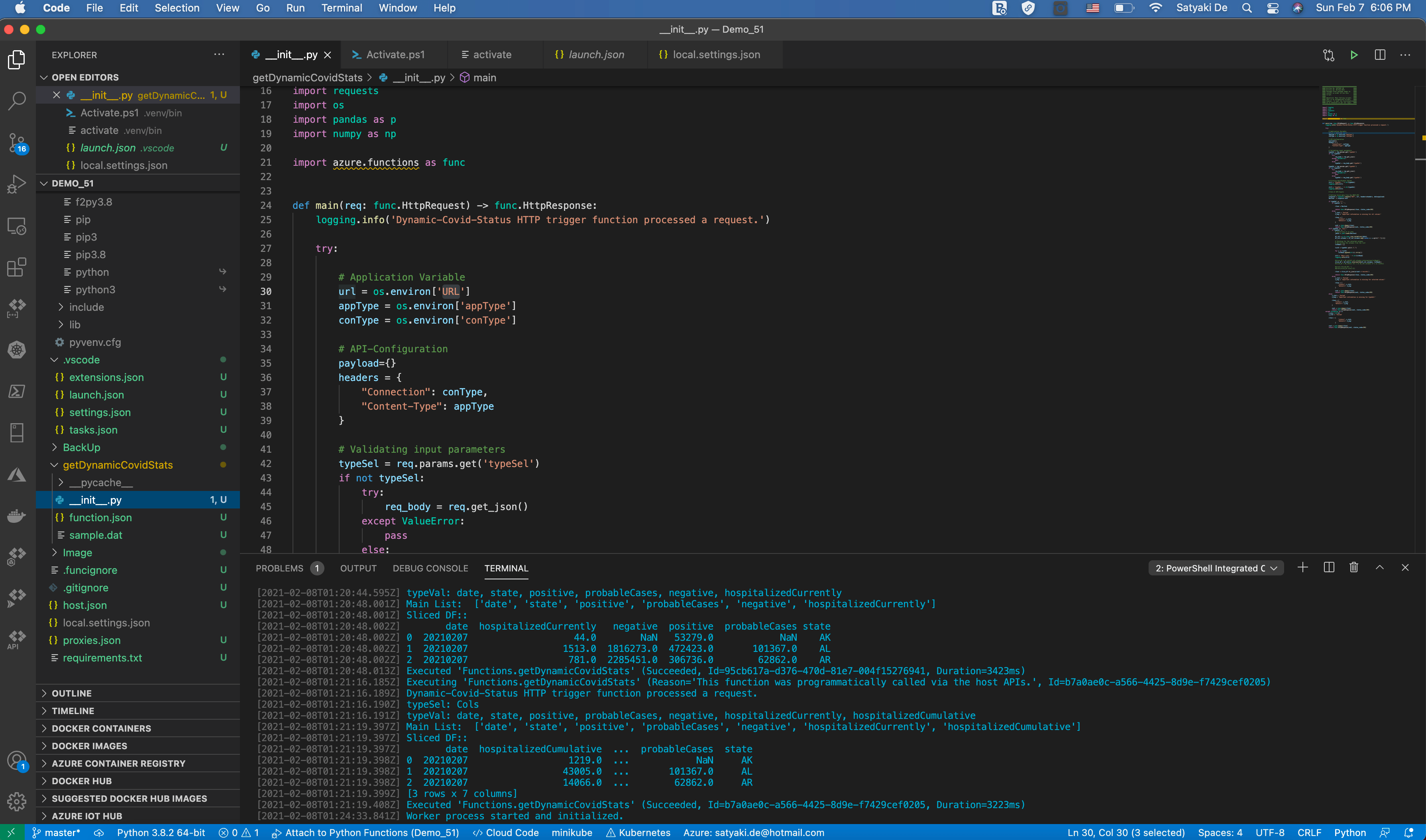Azure function in Visual Studio Code <p value="<amp-fit-text layout="fixed-height" min-font-size="6" max-font-size="72" height="80">Let's test it using Postman -Let’s test it using Postman –

Case 1 (For all the columns):

And, the formatted output is as follows –

Case 2 (For selected columns):

You can find the code in the Github using the following link.

So, finally, we have done it.

I’ll bring some more exciting topic in the coming days from the Python verse.

Till then, Happy Avenging! 😀

Note: All the data & scenario posted here are representational data & scenarios & available over the internet & for educational purpose only.

## Combining the NoSQL(Cosmos DB) & traditional Azure RDBMS in Azure (Time stone solo from Python verse)

Hi Guys!

Today, our main objective is to extend our last post & blending two different kinds of data using Python.

#### What is the Objective?

In this post, our objective is to combine traditional RDBMS from the cloud with Azure’s NO SQL, which is, in this case, is Cosmos DB. And, try to forecast some kind of blended information, which can be aggregated further.

#### Examining Source Data.

##### No SQL Data from Cosmos:

Let’s check one more time the No SQL data created in our last post.

Total, we’ve created 6 records in our last post.

As you can see in red marked areas. From item, one can check the total number of records created. You can also filter out specific record using the Edit Filter blue color button highlighted with blue box & you need to provide the “WHERE CLAUSE” inside it.

##### Azure SQL DB:

Let’s create some data in Azure SQL DB.

But, before that, you need to create SQL DB in the Azure cloud. Here is the official Microsoft link to create DB in Azure. You can refer to it here.

I won’t discuss the detailed steps of creating DB here.

From Azure portal, it looks like –

Let’s see how the data looks like in Azure DB. For our case, we’ll be using the hrMaster DB.

Let’s create the table & some sample data aligned as per our cosmos data.

We will join both the data based on subscriberId & then extract our required columns in our final output.

Good. Now, we’re ready for python scripts.

#### Python Scripts:

In this installment, we’ll be reusing the following python scripts, which is already discussed in my earlier post –

• clsL.py
• clsColMgmt.py
• clsCosmosDBDet.py

So, I’m not going to discuss these scripts.

Before we discuss our scripts, let’s look out the directory structures –

Here is the detailed directory structure between the Windows & MAC O/S.

1. clsConfig.py (This script will create the split csv files or final merge file after the corresponding process. However, this can be used as usual verbose debug logging as well. Hence, the name comes into the picture.)

```##############################################
#### Written By: SATYAKI DE               ####
#### Written On: 25-May-2019              ####
#### Updated On: 02-Jun-2019              ####
####                                      ####
#### Objective: This script is a config   ####
#### file, contains all the keys for      ####
#### azure cosmos db. Application will    ####
#### process these information & perform  ####
#### various CRUD operation on Cosmos DB. ####
##############################################

import os
import platform as pl

class clsConfig(object):
Curr_Path = os.path.dirname(os.path.realpath(__file__))
db_name = 'rnd-de01-usw2-vfa-cdb'
CONTAINER1 = "RealtimeEmail"
CONTAINER3 = "RealtimeHR"

os_det = pl.system()
if os_det == "Windows":
sep = '\\'
else:
sep = '/'

config = {
'SERVER': 'xxxx-xxx.database.windows.net',
'DATABASE_1': 'SalesForceMaster',
'DATABASE_2': 'hrMaster',
'DATABASE_3': 'statMaster',
'DRIVER': '{ODBC Driver 17 for SQL Server}',
'ENV': 'pocdev-saty',
'ENCRYPT_FLAG': "yes",
'TRUST_FLAG': "no",
'TIMEOUT_LIMIT': "30",
'PROCSTAT': "'Y'",
'APP_ID': 1,
'EMAIL_SRC_JSON_FILE': Curr_Path + sep + 'src_file' + sep + 'srcEmail.json',
'HR_SRC_JSON_FILE': Curr_Path + sep + 'src_file' + sep + 'srcHR.json',
'COSMOSDB_ENDPOINT': 'https://rnd-de01-usw2-vfa-cdb.documents.azure.com:443/',
'CONFIG_TABLE': 'ETL_CONFIG_TAB',
'COSMOS_PRIMARYKEY': "XXXXXXXXXXXXXXXXXXXXXXXXXXXXXXXXXXXXXXXXXXXXXXXXXXXXXXXXXXXXIsI00AxKXXXXXgg==",
'ARCH_DIR': Curr_Path + sep + 'arch' + sep,
'COSMOSDB': db_name,
'COSMOS_CONTAINER1': CONTAINER1,
'COSMOS_CONTAINER2': CONTAINER2,
'COSMOS_CONTAINER3': CONTAINER3,
'CONFIG_ORIG': 'Config_orig.csv',
'ENCRYPT_CSV': 'Encrypt_Config.csv',
'DECRYPT_CSV': 'Decrypt_Config.csv',
'PROFILE_PATH': Curr_Path + sep + 'profile' + sep,
'LOG_PATH': Curr_Path + sep + 'log' + sep,
'REPORT_PATH': Curr_Path + sep + 'report',
'APP_DESC_1': 'Feedback Communication',
'DEBUG_IND': 'N',
'INIT_PATH': Curr_Path,
'SQL_QRY_1': "SELECT c.subscriberId, c.sender, c.orderNo, c.orderDate, c.items.orderQty  FROM RealtimeEmail c",
'DB_QRY': "SELECT * FROM c",
'AZURE_SQL_1': "SELECT DISTINCT subscriberId, state, country, annualIncome, customerType FROM dbo.onboardCustomer",
'COLLECTION_QRY': "SELECT * FROM r",
'options': {
'offerThroughput': 1000,
'enableCrossPartitionQuery': True,
'maxItemCount': 2
}
}```

Here, we’ve added a couple of more entries compared to the last time, which points the detailed configuration for Azure SQL DB.

‘SERVER’: ‘xxxx-xxx.database.windows.net’,
‘DATABASE_1’: ‘SalesForceMaster’,
‘DATABASE_2’: ‘hrMaster’,
‘DATABASE_3’: ‘statMaster’,
‘DRIVER’: ‘{ODBC Driver 17 for SQL Server}’,
‘ENV’: ‘pocdev-saty’,
‘ENCRYPT_FLAG’: “yes”,
‘TRUST_FLAG’: “no”,
‘TIMEOUT_LIMIT’: “30”,
‘PROCSTAT’: “‘Y'”,

Here, you need to supply your DB credentials accordingly.

2. clsDBLookup.py (This script will look into the Azure SQL DB & fetch data from the traditional RDBMS of Azure environment.)

```#####################################################
#### Written By: SATYAKI DE                      ####
#### Written On: 25-May-2019                     ####
####                                             ####
#### Objective: This script will check &         ####
#### test the connection with the Azure          ####
#### SQL DB & it will fetch all the records      ####
#### name resied under the same DB of a table.   ####
#####################################################

import pyodbc as py
import pandas as p
from clsConfig import clsConfig as cdc

class clsDBLookup(object):
def __init__(self, lkpTableName = ''):
self.server = cdc.config['SERVER']
self.database = cdc.config['DATABASE_1']
self.database1 = cdc.config['DATABASE_2']
self.database2 = cdc.config['DATABASE_3']
self.driver = cdc.config['DRIVER']
self.env = cdc.config['ENV']
self.encrypt_flg = cdc.config['ENCRYPT_FLAG']
self.trust_flg = cdc.config['TRUST_FLAG']
self.timeout_limit = cdc.config['TIMEOUT_LIMIT']
self.lkpTableName = cdc.config['CONFIG_TABLE']
self.ProcStat = cdc.config['PROCSTAT']
self.AppId = cdc.config['APP_ID']

def LookUpData(self):
try:
# Assigning all the required values
server = self.server
database = self.database1
driver = self.driver
env = self.env
encrypt_flg = self.encrypt_flg
trust_flg = self.trust_flg
timout_limit = self.timeout_limit
lkpTableName = self.lkpTableName
ProcStat = self.ProcStat
AppId = self.AppId

# Creating secure connection
str_conn = 'Driver=' + driver + ';Server=tcp:' + server + ',1433;' \
'Database=' + database + ';Uid=' + username + '@' + env + ';' \
'Pwd=' + password + ';Encrypt=' + encrypt_flg + ';' \
'TrustServerCertificate=' + trust_flg + ';Connection Timeout=' + timout_limit + ';'

db_con_azure = py.connect(str_conn)

query = " SELECT [ruleId] as ruleId, [ruleName] as ruleName, [ruleSQL] as ruleSQL, " \
" [ruleFlag] as ruleFlag, [appId] as appId, [DBType] as DBType, " \
" [DBName] as DBName FROM [dbo][" + lkpTableName + "] WHERE ruleFLag = " + ProcStat + " " \
" and appId = " + AppId + " ORDER BY ruleId "

# Closing the connection
db_con_azure.close()

return df
except Exception as e:
x = str(e)
print(x)
df = p.DataFrame()

return df

try:
# Assigning all the required values
server = self.server
database = self.database1
driver = self.driver
env = self.env
encrypt_flg = self.encrypt_flg
trust_flg = self.trust_flg
timout_limit = self.timeout_limit
lkpTableName = self.lkpTableName
ProcStat = self.ProcStat
AppId = self.AppId

# Creating secure connection
str_conn = 'Driver=' + driver + ';Server=tcp:' + server + ',1433;' \
'Database=' + database + ';Uid=' + username + '@' + env + ';' \
'Pwd=' + password + ';Encrypt=' + encrypt_flg + ';' \
'TrustServerCertificate=' + trust_flg + ';Connection Timeout=' + timout_limit + ';'

# print("Connection Details:: ", str_conn)
db_con_azure = py.connect(str_conn)

query = sql

# Closing the connection
db_con_azure.close()

return df
except Exception as e:
x = str(e)
print(x)
df = p.DataFrame()

return df```

Major lines to discuss –

`azure_sqldb_read(self, sql):`

Getting the source SQL supplied from the configuration script.

```db_con_azure = py.connect(str_conn)

query = sql

After creating a successful connection, our application will read the SQL & fetch the data & store that into a pandas dataframe and return the output to the primary calling function.

3. callCosmosAPI.py (This is the main script, which will call all the methods to blend the data. Hence, the name comes into the picture.)

```##############################################
#### Written By: SATYAKI DE               ####
#### Written On: 25-May-2019              ####
#### Modified On 02-Jun-2019              ####
####                                      ####
#### Objective: Main calling scripts.     ####
##############################################

import clsColMgmt as cm
import clsCosmosDBDet as cmdb
from clsConfig import clsConfig as cf
import pandas as p
import clsLog as cl
import logging
import datetime
import json
import clsDBLookup as dbcon

# Disbling Warning
def warn(*args, **kwargs):
pass

import warnings
warnings.warn = warn

def getDate(row):
try:
d1 = row['orderDate']
d1_str = str(d1)
d1_dt_part, sec = d1_str.split('.')
dt_part1 = d1_dt_part.replace('T', ' ')

return dt_part1
except Exception as e:
x = str(e)
print(x)
dt_part1 = ''

return dt_part1

# Lookup functions from
# Azure cloud SQL DB

var = datetime.datetime.now().strftime("%Y-%m-%d_%H-%M-%S")

def main():
try:
df_ret = p.DataFrame()
df_ret_2 = p.DataFrame()
df_ret_2_Mod = p.DataFrame()

debug_ind = 'Y'

# Initiating Log Class
l = cl.clsLog()

general_log_path = str(cf.config['LOG_PATH'])

# Enabling Logging Info
logging.basicConfig(filename=general_log_path + 'consolidated.log', level=logging.INFO)

# Moving previous day log files to archive directory
arch_dir = cf.config['ARCH_DIR']
log_dir = cf.config['LOG_PATH']

print("Archive Directory:: ", arch_dir)
print("Log Directory::", log_dir)

print("*" * 157)
print("Testing COSMOS DB Connection!")
print("*" * 157)

# Checking Cosmos DB Azure
y = cmdb.clsCosmosDBDet()
ret_val = y.test_db_con()

if ret_val == 0:
print()
print("Cosmos DB Connection Successful!")
print("*" * 157)
else:
print()
print("Cosmos DB Connection Failure!")
print("*" * 157)
raise Exception

print("*" * 157)

# Accessing from Azure SQL DB
x1 = dbcon.clsDBLookup()

print("Azure SQL DB::")
print(act_df)
print()

print("-" * 157)

# Calling the function 1
print("RealtimeEmail::")

# Fetching First collection data to dataframe
print("Fethcing Comos Collection Data!")

sql_qry_1 = cf.config['SQL_QRY_1']
msg = "Documents generatd based on unique key"
collection_flg = 1

x = cm.clsColMgmt()
df_ret = x.fetch_data(sql_qry_1, msg, collection_flg)

l.logr('1.EmailFeedback_' + var + '.csv', debug_ind, df_ret, 'log')
print('RealtimeEmail Data::')
print(df_ret)
print()

# Checking execution status
ret_val = int(df_ret.shape)

if ret_val == 0:
print("*" * 157)
else:
print("Successfully fetched!")
print("*" * 157)

# Calling the 2nd Collection

# Fetching First collection data to dataframe
print("Fethcing Cosmos Collection Data!")

# Query using parameters
sql_qry_2 = cf.config['SQL_QRY_2']
msg_2 = "Documents generated based on RealtimeTwitterFeedback feed!"
collection_flg = 2

val = 'crazyGo'
param_det = [{"name": "@CrVal", "value": val}]

x1 = cm.clsColMgmt()
df_ret_2 = x1.fetch_data(sql_qry_2, msg_2, collection_flg, add_param, param_det)

l.logr('2.TwitterFeedback_' + var + '.csv', debug_ind, df_ret, 'log')
print(df_ret_2)
print()

# Checking execution status
ret_val_2 = int(df_ret_2.shape)

if ret_val_2 == 0:
print("*" * 157)
else:
print("Successfuly row feteched!")
print("*" * 157)

# Merging NoSQL Data (Cosmos DB) with Relational DB (Azure SQL DB)
df_Fin_temp = p.merge(df_ret, act_df, on='subscriberId', how='inner')

df_fin = df_Fin_temp[['orderDate', 'orderNo', 'sender', 'state', 'country', 'customerType']]

print("Initial Combined Data (From Cosmos & Azure SQL DB) :: ")
print(df_fin)

l.logr('3.InitCombine_' + var + '.csv', debug_ind, df_fin, 'log')

# Transforming the orderDate as per standard format
df_fin['orderDateM'] = df_fin.apply(lambda row: getDate(row), axis=1)

# Dropping the old column & renaming the new column to old column
df_fin.drop(columns=['orderDate'], inplace=True)
df_fin.rename(columns={'orderDateM': 'orderDate'}, inplace=True)

print("*" * 157)
print()
print("Final Combined & Transformed result:: ")
print(df_fin)

l.logr('4.Final_Combine_' + var + '.csv', debug_ind, df_fin, 'log')
print("*" * 157)

except ValueError:
print("No relevant data to proceed!")

except Exception as e:
print("Top level Error: args:{0}, message{1}".format(e.args, e.message))

if __name__ == "__main__":
main()```

The key lines from this script –

```def getDate(row):
try:
d1 = row['orderDate']
d1_str = str(d1)
d1_dt_part, sec = d1_str.split('.')
dt_part1 = d1_dt_part.replace('T', ' ')

return dt_part1
except Exception as e:
x = str(e)
print(x)
dt_part1 = ''

return dt_part1```

This function converts NoSQL date data type more familiar format.

##### Transformed Date:
```# Accessing from Azure SQL DB
x1 = dbcon.clsDBLookup()

print("Azure SQL DB::")
print(act_df)
print()```

Above lines are calling the Azure SQL DB method to retrieve the RDBMS data into our dataframe.

```# Merging NoSQL Data (Cosmos DB) with Relational DB (Azure SQL DB)
df_Fin_temp = p.merge(df_ret, act_df, on='subscriberId', how='inner')

df_fin = df_Fin_temp[['orderDate', 'orderNo', 'sender', 'state', 'country', 'customerType']]```

In these above lines, we’re joining the data retrieved from two different kinds of the database to prepare our initial combined dataframe. Also, we’ve picked only the desired column, which will be useful for us.

```# Transforming the orderDate as per standard format
df_fin['orderDateM'] = df_fin.apply(lambda row: getDate(row), axis=1)

# Dropping the old column & renaming the new column to old column
df_fin.drop(columns=['orderDate'], inplace=True)
df_fin.rename(columns={'orderDateM': 'orderDate'}, inplace=True)```

In the above lines, we’re transforming our date field, as shown above in one of our previous images by calling the getDate method.

Let’s see the directory structure of our program –

Let’s see how it looks when it runs –

Windows:

MAC:

So, finally, we’ve successfully blended the data & make more meaningful data projection.

Following python packages are required to run this application –

pip install azure

pip install azure-cosmos

pip install pandas

pip install requests

pip install pyodbc

This application tested on Python3.7.1 & Python3.7.2 as well. As per Microsoft, their official supported version is Python3.5.

I hope you’ll like this effort.

Wait for the next installment. Till then, Happy Avenging. 😀

[Note: All the sample data are available/prepared in the public domain for research & study.]

## Improvement of Pandas data processing performance using Multi-threading with the Queue (Another crossover of Space Stone, Reality Stone & Power Stone)

Today, we’ll discuss how to improve your panda’s data processing power using Multi-threading. Note that, we are not going to use any third party python package. Also, we’ll be using a couple of python scripts, which we’ve already discussed in our previous posts. Hence, this time, I won’t post them here.

Please refer the following scripts –

```a. callClient.py
b. callRunServer.py
c. clsConfigServer.py
d. clsEnDec.py
f. clsL.py
g. clsParam.py
h. clsSerial.py
i. clsWeb.py```

Please find the above scripts described here with details.

So, today, we’ll be looking into how the multi-threading really helps the application to gain some performance over others.

Let’s go through our existing old sample files –And, we’ve four columns that are applicable for encryption. This file contains 10K records. That means the application will make 40K calls to the server for a different kind of encryption for each column.

Now, if you are going with the serial approach, which I’ve already discussed here, will take significant time for data processing. However, if we could club a few rows as one block & in this way we can create multiple blocks out of our data csv like this –As you can see that blocks are marked with a different color. So, now if you send each block of data in parallel & send the data for encryption. Ideally, you will be able to process data much faster than the usual serial process. And, this what we would be looking for with the help of python’s multi-threading & queue. Without the queue, this program won’t be possible as the queue maintains the data & process integrity.

One more thing we would like to explain here. Whenever this application is sending the block of data. It will be posting that packed into a (key, value) dictionary randomly. Key will be the thread name. The reason, we’re not expecting data after process might arrive in some random order wrapped with the dictionary as well. Once the application received all the dictionary with dataframe with encrypted/decrypted data, the data will be rearranged based on the key & then joined back with the rest of the data.

Let’s see one sample way of sending & receiving random thread –The left-hand side, the application is splitting the recordset into small chunks of a group. Once, those group created, using python multi-threading the application is now pushing them into the queue for the producer to produce the encrypted/decrypted value. Similar way, after processing the application will push the final product into the queue for consuming the final output.

This is the pictorial representation of dictionary ordering based on the key-value & then the application will extract the entire data to form the target csv file.Let’s explore the script –

1. clsParallel.py (This script will consume the split csv files & send the data blocks in the form of the dictionary using multi-threading to the API for encryption in parallel. Hence, the name comes into the picture.)

 ``` 1 2 3 4 5 6 7 8 9 10 11 12 13 14 15 16 17 18 19 20 21 22 23 24 25 26 27 28 29 30 31 32 33 34 35 36 37 38 39 40 41 42 43 44 45 46 47 48 49 50 51 52 53 54 55 56 57 58 59 60 61 62 63 64 65 66 67 68 69 70 71 72 73 74 75 76 77 78 79 80 81 82 83 84 85 86 87 88 89 90 91 92 93 94 95 96 97 98 99 100 101 102 103 104 105 106 107 108 109 110 111 112 113 114 115 116 117 118 119 120 121 122 123 124 125 126 127 128 129 130 131 132 133 134 135 136 137 138 139 140 141 142 143 144 145 146 147 148 149 150 151 152 153 154 155 156 157 158 159 160 161 162 163 164 165 166 167 168 169 170 171 172 173 174 175 176 177 178 179 180 181 182 183 184 185 186 187 188 189 190 191 192 193 194 195 196 197 198 199 200 201 202 203 204 205 206 207 208 209 210 211 212 213 214 215 216 217 218 219 220 221 222 223 224 225 226 227 228 229 230 231 232 233 234 235 236 237 238 239 240 241 242 243 244 245 246 247 248 249 250 251 252 253 254 255 256 257 258 259 260 261 262 263 264 265 266 267 268 269 270 271 272 273 274 275 276 277 278 279 280 281 282 283 284 285 286 287 288 289 290 291 292 293 294 295 296 297 298 299 300 301 302 303 304 305 306 307 308 309 310 311 312 313 314 315 316 317 318 319 320 321 322 323 324 325 326 327 328 329 330 331 332 333 334 335 336 337 338 339 340 341 342 343 344 345 346 347 348 349 350 351 352 353 354 355 356 357 358 359 360 361 362 363 364 365 366 367 368 369 370 371 372 373 374 375 376 377 378 379 380 381 382 383 384 385 386 387 388 389 390 391 392 393 394 395 396 397 398 399 400 401 402 403 404 405 406 407 408 409 410 411 412 413 414 415 416 417 418 419 420 421 422 423 424 425 426 427 428 429 430 431 432 433 434 435 436 437 438 439 440 441 442 443 444 445 446 447 448 449 450 451 452 453 454 455 456 457 458 459 460 461 462 463 464 465 466 467 468 469 470 471 472 473 474 475 476 477 478 479 480 481 482 483 484 485 486 487 488 489 490 491 492 493 494 495 496 497 498 499 500 501 502 503 504 505 506 507 508 509 510 511 512 513 514 515 516 517 518 519``` ```import pandas as p import clsWeb as cw import datetime from clsParam import clsParam as cf import threading from queue import Queue import gc import signal import time import os # Declaring Global Variable q = Queue() m = Queue() tLock = threading.Lock() threads = [] fin_dict = {} fin_dict_1 = {} stopping = threading.Event() # Disbling Warnings def warn(*args, **kwargs): pass import warnings warnings.warn = warn class clsParallel(object): def __init__(self): self.path = cf.config['PATH'] self.EncryptMode = str(cf.config['ENCRYPT_MODE']) self.DecryptMode = str(cf.config['DECRYPT_MODE']) self.num_worker_threads = int(cf.config['NUM_OF_THREAD']) # Lookup Methods for Encryption def encrypt_acctNbr(self, row): # Declaring Local Variable en_AcctNbr = '' json_source_str = '' # Capturing essential values EncryptMode = self.EncryptMode lkp_acctNbr = row['Acct_Nbr'] str_acct_nbr = str(lkp_acctNbr) fil_acct_nbr = str_acct_nbr.strip() # Forming JSON String for this field json_source_str = '{"dataGroup":"GrDet","data":"' + fil_acct_nbr + '","dataTemplate":"subGrAcct_Nbr"}' # Identifying Length of the field len_acct_nbr = len(fil_acct_nbr) # This will trigger the service if it has valid data if len_acct_nbr > 0: x = cw.clsWeb(json_source_str) en_AcctNbr = x.getResponse(EncryptMode) else: en_AcctNbr = '' return en_AcctNbr def encrypt_Name(self, row): # Declaring Local Variable en_AcctName = '' # Capturing essential values EncryptMode = self.EncryptMode lkp_acctName = row['Name'] str_acct_name = str(lkp_acctName) fil_acct_name = str_acct_name.strip() # Forming JSON String for this field json_source_str = '{"dataGroup":"GrDet","data":"' + fil_acct_name + '","dataTemplate":"subGrName"}' # Identifying Length of the field len_acct_nbr = len(fil_acct_name) # This will trigger the service if it has valid data if len_acct_nbr > 0: x = cw.clsWeb(json_source_str) en_AcctName = x.getResponse(EncryptMode) else: en_AcctName = '' return en_AcctName def encrypt_Phone(self, row): # Declaring Local Variable en_Phone = '' # Capturing essential values EncryptMode = self.EncryptMode lkp_phone = row['Phone'] str_phone = str(lkp_phone) fil_phone = str_phone.strip() # Forming JSON String for this field json_source_str = '{"dataGroup":"GrDet","data":"' + fil_phone + '","dataTemplate":"subGrPhone"}' # Identifying Length of the field len_acct_nbr = len(fil_phone) # This will trigger the service if it has valid data if len_acct_nbr > 0: x = cw.clsWeb(json_source_str) en_Phone = x.getResponse(EncryptMode) else: en_Phone = '' return en_Phone def encrypt_Email(self, row): # Declaring Local Variable en_Email = '' # Capturing essential values EncryptMode = self.EncryptMode lkp_email = row['Email'] str_email = str(lkp_email) fil_email = str_email.strip() # Forming JSON String for this field json_source_str = '{"dataGroup":"GrDet","data":"' + fil_email + '","dataTemplate":"subGrEmail"}' # Identifying Length of the field len_acct_nbr = len(fil_email) # This will trigger the service if it has valid data if len_acct_nbr > 0: x = cw.clsWeb(json_source_str) en_Email = x.getResponse(EncryptMode) else: en_Email = '' return en_Email # Lookup Methods for Decryption def decrypt_acctNbr(self, row): # Declaring Local Variable de_AcctNbr = '' json_source_str = '' # Capturing essential values EncryptMode = self.DecryptMode lkp_acctNbr = row['Acct_Nbr'] str_acct_nbr = str(lkp_acctNbr) fil_acct_nbr = str_acct_nbr.strip() # Forming JSON String for this field json_source_str = '{"dataGroup":"GrDet","data":"' + fil_acct_nbr + '","dataTemplate":"subGrAcct_Nbr"}' # Identifying Length of the field len_acct_nbr = len(fil_acct_nbr) # This will trigger the service if it has valid data if len_acct_nbr > 0: x = cw.clsWeb(json_source_str) de_AcctNbr = x.getResponse(EncryptMode) else: de_AcctNbr = '' return de_AcctNbr def decrypt_Name(self, row): # Declaring Local Variable de_AcctName = '' # Capturing essential values EncryptMode = self.DecryptMode lkp_acctName = row['Name'] str_acct_name = str(lkp_acctName) fil_acct_name = str_acct_name.strip() # Forming JSON String for this field json_source_str = '{"dataGroup":"GrDet","data":"' + fil_acct_name + '","dataTemplate":"subGrName"}' # Identifying Length of the field len_acct_nbr = len(fil_acct_name) # This will trigger the service if it has valid data if len_acct_nbr > 0: x = cw.clsWeb(json_source_str) de_AcctName = x.getResponse(EncryptMode) else: de_AcctName = '' return de_AcctName def decrypt_Phone(self, row): # Declaring Local Variable de_Phone = '' # Capturing essential values EncryptMode = self.DecryptMode lkp_phone = row['Phone'] str_phone = str(lkp_phone) fil_phone = str_phone.strip() # Forming JSON String for this field json_source_str = '{"dataGroup":"GrDet","data":"' + fil_phone + '","dataTemplate":"subGrPhone"}' # Identifying Length of the field len_acct_nbr = len(fil_phone) # This will trigger the service if it has valid data if len_acct_nbr > 0: x = cw.clsWeb(json_source_str) de_Phone = x.getResponse(EncryptMode) else: de_Phone = '' return de_Phone def decrypt_Email(self, row): # Declaring Local Variable de_Email = '' # Capturing essential values EncryptMode = self.DecryptMode lkp_email = row['Email'] str_email = str(lkp_email) fil_email = str_email.strip() # Forming JSON String for this field json_source_str = '{"dataGroup":"GrDet","data":"' + fil_email + '","dataTemplate":"subGrEmail"}' # Identifying Length of the field len_acct_nbr = len(fil_email) # This will trigger the service if it has valid data if len_acct_nbr > 0: x = cw.clsWeb(json_source_str) de_Email = x.getResponse(EncryptMode) else: de_Email = '' return de_Email def getEncrypt(self, df_dict): try: df_input = p.DataFrame() df_fin = p.DataFrame() # Assigning Target File Basic Name for k, v in df_dict.items(): Thread_Name = k df_input = v # Checking total count of rows count_row = int(df_input.shape) # print('Part number of records to process:: ', count_row) if count_row > 0: # Deriving rows df_input['Encrypt_Acct_Nbr'] = df_input.apply(lambda row: self.encrypt_acctNbr(row), axis=1) df_input['Encrypt_Name'] = df_input.apply(lambda row: self.encrypt_Name(row), axis=1) df_input['Encrypt_Phone'] = df_input.apply(lambda row: self.encrypt_Phone(row), axis=1) df_input['Encrypt_Email'] = df_input.apply(lambda row: self.encrypt_Email(row), axis=1) # Dropping original columns df_input.drop(['Acct_Nbr', 'Name', 'Phone', 'Email'], axis=1, inplace=True) # Renaming new columns with the old column names df_input.rename(columns={'Encrypt_Acct_Nbr':'Acct_Nbr'}, inplace=True) df_input.rename(columns={'Encrypt_Name': 'Name'}, inplace=True) df_input.rename(columns={'Encrypt_Phone': 'Phone'}, inplace=True) df_input.rename(columns={'Encrypt_Email': 'Email'}, inplace=True) # New Column List Orders column_order = ['Acct_Nbr', 'Name', 'Acct_Addr_1', 'Acct_Addr_2', 'Phone', 'Email', 'Serial_No'] df_fin = df_input.reindex(column_order, axis=1) fin_dict[Thread_Name] = df_fin return 0 except Exception as e: df_error = p.DataFrame({'Acct_Nbr':str(e), 'Name':'', 'Acct_Addr_1':'', 'Acct_Addr_2':'', 'Phone':'', 'Email':'', 'Serial_No':''}) fin_dict[Thread_Name] = df_error return 1 def getEncryptWQ(self): item_dict = {} item = '' while True: try: #item_dict = q.get() item_dict = q.get_nowait() for k, v in item_dict.items(): # Assigning Target File Basic Name item = str(k) if ((item == 'TEND') | (item == '')): break if ((item != 'TEND') | (item != '')): self.getEncrypt(item_dict) q.task_done() except Exception: break def getEncryptParallel(self, df_payload): start_pos = 0 end_pos = 0 l_dict = {} c_dict = {} min_val_list = {} cnt = 0 num_worker_threads = self.num_worker_threads split_df = p.DataFrame() df_ret = p.DataFrame() # Assigning Target File Basic Name df_input = df_payload # Checking total count of rows count_row = df_input.shape print('Total number of records to process:: ', count_row) interval = int(count_row / num_worker_threads) + 1 actual_worker_task = int(count_row / interval) + 1 for i in range(actual_worker_task): t = threading.Thread(target=self.getEncryptWQ) t.start() threads.append(t) name = str(t.getName()) if ((start_pos + interval) < count_row): end_pos = start_pos + interval else: end_pos = start_pos + (count_row - start_pos) split_df = df_input.iloc[start_pos:end_pos] l_dict[name] = split_df if ((start_pos > count_row) | (start_pos == count_row)): break else: start_pos = start_pos + interval q.put(l_dict) cnt += 1 # block until all tasks are done q.join() # stop workers for i in range(actual_worker_task): c_dict['TEND'] = p.DataFrame() q.put(c_dict) for t in threads: t.join() for k, v in fin_dict.items(): min_val_list[int(k.replace('Thread-',''))] = v min_val = min(min_val_list, key=int) for k, v in sorted(fin_dict.items(), key=lambda k:int(k.replace('Thread-',''))): if int(k.replace('Thread-','')) == min_val: df_ret = fin_dict[k] else: d_frames = [df_ret, fin_dict[k]] df_ret = p.concat(d_frames) # Releasing Memory del[[split_df]] gc.collect() return df_ret def getDecrypt(self, df_encrypted_dict): try: df_input = p.DataFrame() df_fin = p.DataFrame() # Assigning Target File Basic Name for k, v in df_encrypted_dict.items(): Thread_Name = k df_input = v # Checking total count of rows count_row = int(df_input.shape) if count_row > 0: # Deriving rows df_input['Decrypt_Acct_Nbr'] = df_input.apply(lambda row: self.decrypt_acctNbr(row), axis=1) df_input['Decrypt_Name'] = df_input.apply(lambda row: self.decrypt_Name(row), axis=1) df_input['Decrypt_Phone'] = df_input.apply(lambda row: self.decrypt_Phone(row), axis=1) df_input['Decrypt_Email'] = df_input.apply(lambda row: self.decrypt_Email(row), axis=1) # Dropping original columns df_input.drop(['Acct_Nbr', 'Name', 'Phone', 'Email'], axis=1, inplace=True) # Renaming new columns with the old column names df_input.rename(columns={'Decrypt_Acct_Nbr':'Acct_Nbr'}, inplace=True) df_input.rename(columns={'Decrypt_Name': 'Name'}, inplace=True) df_input.rename(columns={'Decrypt_Phone': 'Phone'}, inplace=True) df_input.rename(columns={'Decrypt_Email': 'Email'}, inplace=True) # New Column List Orders column_order = ['Acct_Nbr', 'Name', 'Acct_Addr_1', 'Acct_Addr_2', 'Phone', 'Email'] df_fin = df_input.reindex(column_order, axis=1) fin_dict_1[Thread_Name] = df_fin return 0 except Exception as e: df_error = p.DataFrame({'Acct_Nbr': str(e), 'Name': '', 'Acct_Addr_1': '', 'Acct_Addr_2': '', 'Phone': '', 'Email': ''}) fin_dict_1[Thread_Name] = df_error return 1 def getDecryptWQ(self): item_dict = {} item = '' while True: try: #item_dict = q.get() item_dict = m.get_nowait() for k, v in item_dict.items(): # Assigning Target File Basic Name item = str(k) if ((item == 'TEND') | (item == '')): return True #break if ((item != 'TEND') | (item != '')): self.getDecrypt(item_dict) m.task_done() except Exception: break def getDecryptParallel(self, df_payload): start_pos = 0 end_pos = 0 l_dict_1 = {} c_dict_1 = {} cnt = 0 num_worker_threads = self.num_worker_threads split_df = p.DataFrame() df_ret_1 = p.DataFrame() min_val_list = {} # Assigning Target File Basic Name df_input_1 = df_payload # Checking total count of rows count_row = df_input_1.shape print('Total number of records to process:: ', count_row) interval = int(count_row / num_worker_threads) + 1 actual_worker_task = int(count_row / interval) + 1 for i in range(actual_worker_task): t_1 = threading.Thread(target=self.getDecryptWQ) t_1.start() threads.append(t_1) name = str(t_1.getName()) if ((start_pos + interval) < count_row): end_pos = start_pos + interval else: end_pos = start_pos + (count_row - start_pos) split_df = df_input_1.iloc[start_pos:end_pos] l_dict_1[name] = split_df if ((start_pos > count_row) | (start_pos == count_row)): break else: start_pos = start_pos + interval m.put(l_dict_1) cnt += 1 # block until all tasks are done m.join() # stop workers for i in range(actual_worker_task): c_dict_1['TEND'] = p.DataFrame() m.put(c_dict_1) for t_1 in threads: t_1.join() for k, v in fin_dict_1.items(): min_val_list[int(k.replace('Thread-',''))] = v min_val = min(min_val_list, key=int) for k, v in sorted(fin_dict_1.items(), key=lambda k:int(k.replace('Thread-',''))): if int(k.replace('Thread-','')) == min_val: df_ret_1 = fin_dict_1[k] else: d_frames = [df_ret_1, fin_dict_1[k]] df_ret_1 = p.concat(d_frames) # Releasing Memory del[[split_df]] gc.collect() return df_ret_1 ```

Let’s explain the key snippet from the code. For your information, we’re not going to describe all the encryption methods such as –

# Encryption Method
encrypt_acctNbr

encrypt_Name
encrypt_Phone
encrypt_Email

# Decryption Method
decrypt_acctNbr
decrypt_Name
decrypt_Phone
decrypt_Email

As we’ve already described the logic of these methods in our previous post.

```# Checking total count of rows
count_row = df_input.shape
print('Total number of records to process:: ', count_row)

interval = int(count_row / num_worker_threads) + 1
actual_worker_task = int(count_row / interval) + 1```

Fetching the total number of rows from the dataframe. Based on the row count, the application will derive the actual number of threads that will be used for parallelism.

```for i in range(actual_worker_task):
t.start()
name = str(t.getName())

if ((start_pos + interval) < count_row):
end_pos = start_pos + interval
else:
end_pos = start_pos + (count_row - start_pos)

split_df = df_input.iloc[start_pos:end_pos]
l_dict[name] = split_df

if ((start_pos > count_row) | (start_pos == count_row)):
break
else:
start_pos = start_pos + interval

q.put(l_dict)
cnt += 1```

Here, the application is splitting the data into multiple groups of smaller data packs & then combining them into (key, value) dictionary & finally placed them into the individual queue.

```# block until all tasks are done
q.join()```

This will join the queue process. This will ensure that queues are free after consuming the data.

```# stop workers
c_dict['TEND'] = p.DataFrame()
q.put(c_dict)

t.join()```

The above lines are essential. As this will help the process to identify that no more data are left to send at the queue. And, the main thread will wait until all the threads are done.

```for k, v in fin_dict.items():

min_val = min(min_val_list, key=int)```

Once, all the jobs are done. The application will find the minimum thread value & based on that we can sequence all the data chunks as explained in our previous image & finally clubbed them together to form the complete csv.

```for k, v in sorted(fin_dict.items(), key=lambda k:int(k.replace('Thread-',''))):
df_ret = fin_dict[k]
else:
d_frames = [df_ret, fin_dict[k]]
df_ret = p.concat(d_frames)```

As already explained, using the starting point of our data dictionary element, the application is clubbing the data back to the main csv.

Next method, which we’ll be explaining is –

`getEncryptWQ`

Please find the key lines –

```while True:
try:
#item_dict = q.get()
item_dict = q.get_nowait()

for k, v in item_dict.items():
# Assigning Target File Basic Name
item = str(k)

if ((item == 'TEND') | (item == '')):
break

if ((item != 'TEND') | (item != '')):
self.getEncrypt(item_dict)

except Exception:
break```

This method will consume the data & processing it for encryption or decryption. This will continue to do the work until or unless it receives the key value as TEND or the queue is empty.

Let’s compare the statistics between Windows & MAC.

Let’s see the file structure first –

##### Windows (16 GB – Core 2) Vs Mac (10 GB – Core 2):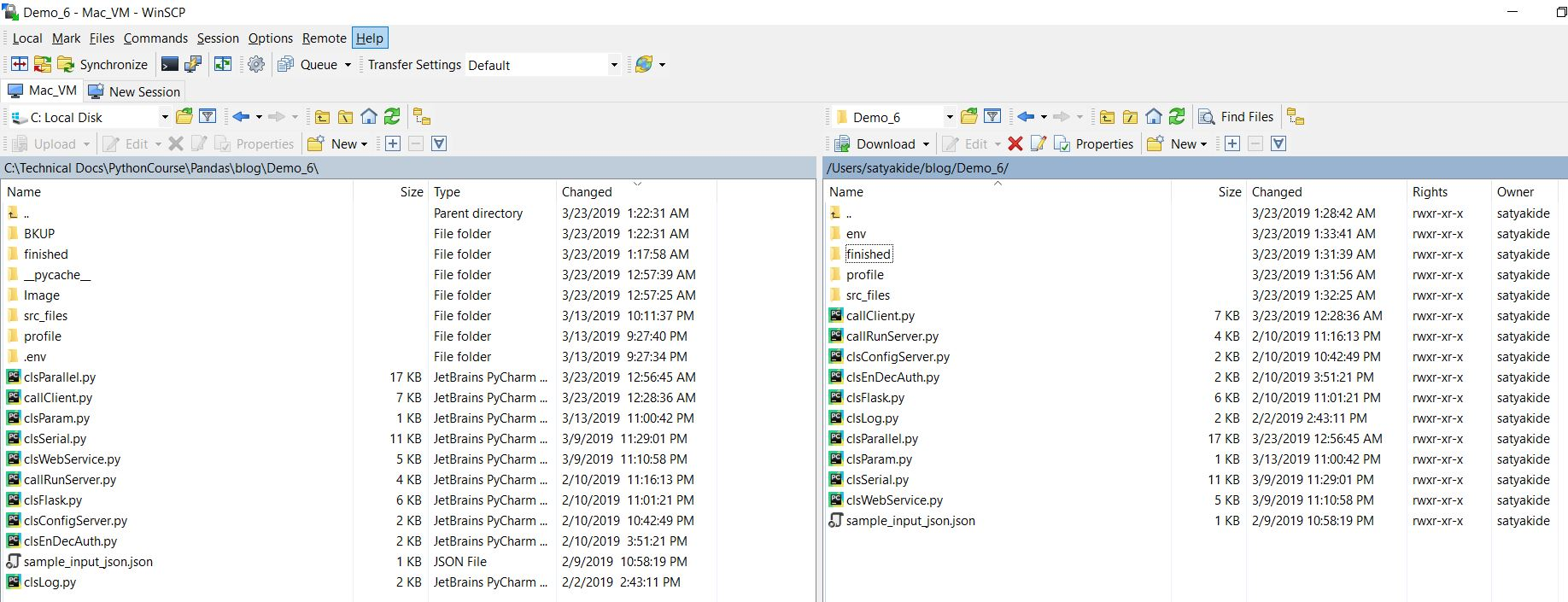##### Windows (16 GB – Core 2):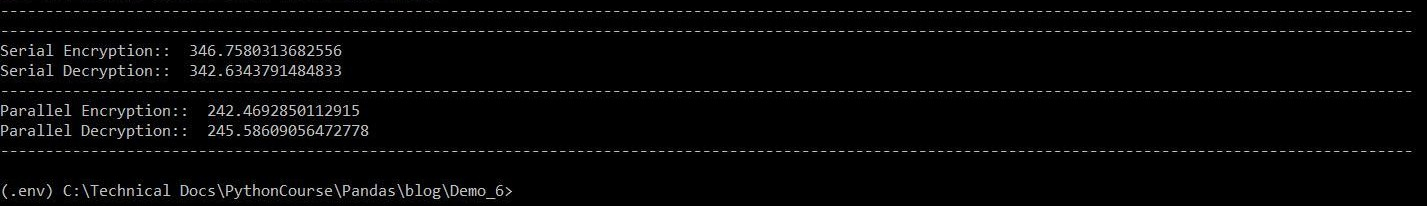##### Mac (10 GB – Core 2):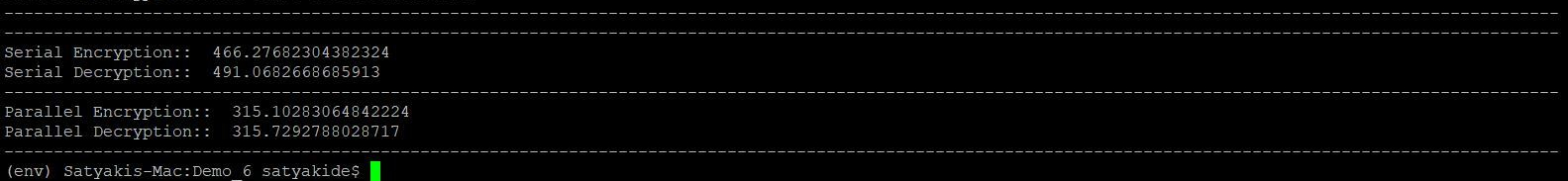##### Windows (16 GB – Core 2):##### Mac (10 GB – Core 2):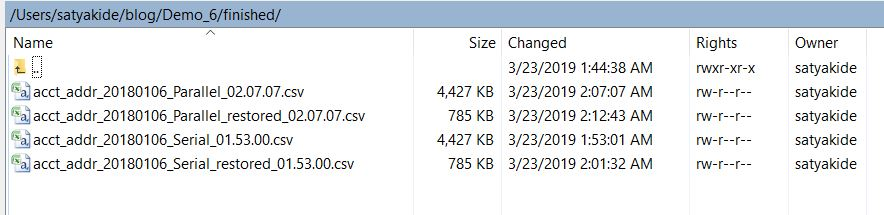Here is the final output –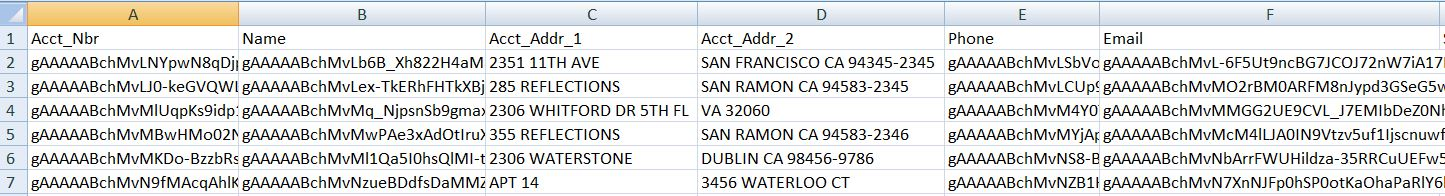So, we’ve achieved our target goal.

I’ll be back with another installment from the Python verse.

Till then – Happy Avenging!

## Encryption/Decryption, JSON, API, Flask Framework in Python (Crossover between Reality Stone & Time Stone in Python Verse)

Hi Guys,

Today, we’ll be looking into another exciting installment of cross-over between Reality Stone & Timestone from the python verse.

We’ll be exploring Encryption/Decryption implemented using the Flask Framework Server component. We would like to demonstrate this Encrypt/Decrypt features as Server API & then we can call it from clients like Postman to view the response.

So, here are primary focus will be implementing this in Server-side rather than the client-side.

However, there is a catch. We would like to implement different kind of encryption or decryption based on our source data.

Let’s look into the sample data first –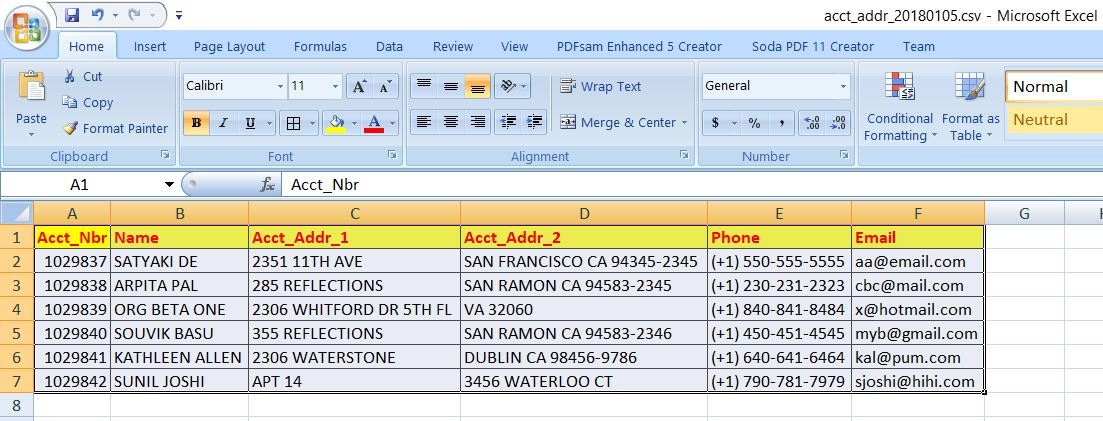As you can see, we intend to encrypt Account Number encryption with different salt compared to Name or Phone or Email. Hence, we would be using different salt to encrypt our sample data & get the desired encrypt/decrypt output.

From the above data, we can create the following types of JSON payload –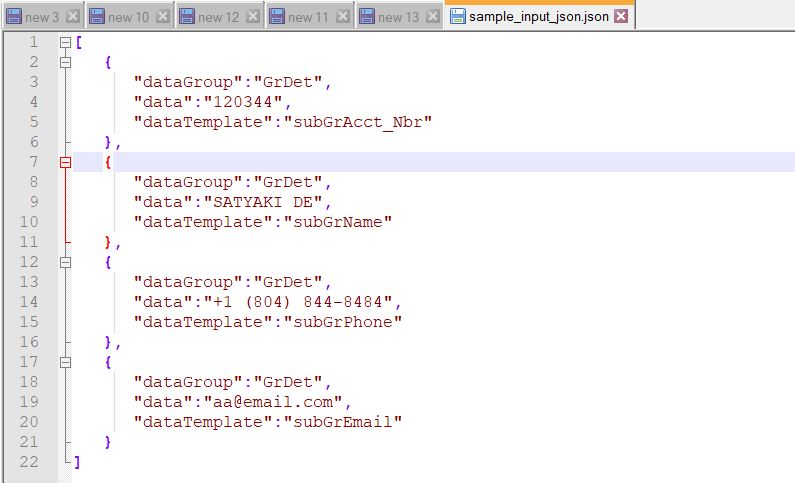Let’s explore –

Before we start, we would like to show you the directory structure of Windows & MAC as we did the same in my earlier post as well.Following are the scripts that we’re using to develop this server applications & they are as follows –

1. clsConfigServer.py (This script contains all the parameters of the server.)

 ``` 1 2 3 4 5 6 7 8 9 10 11 12 13 14 15 16 17 18 19 20 21 22 23 24 25 26 27 28 29 30 31 32 33 34 35 36 37 38 39 40``` ```########################################### #### Written By: SATYAKI DE ######## #### Written On: 10-Feb-2019 ######## #### ######## #### Objective: Parameter File ######## ########################################### import os import platform as pl # Checking with O/S system os_det = pl.system() class clsConfigServer(object): Curr_Path = os.path.dirname(os.path.realpath(__file__)) if os_det == "Windows": config = { 'FILE': 'acct_addr_20180112.csv', 'SRC_FILE_PATH': Curr_Path + '\\' + 'src_file\\', 'PROFILE_FILE_PATH': Curr_Path + '\\' + 'profile\\', 'HOST_IP_ADDR': '0.0.0.0', 'DEF_SALT': 'iooquzKtqLwUwXG3rModqj_fIl409vemWg9PekcKh2o=', 'ACCT_NBR_SALT': 'iooquzKtqLwUwXG3rModqj_fIlpp1vemWg9PekcKh2o=', 'NAME_SALT': 'iooquzKtqLwUwXG3rModqj_fIlpp1026Wg9PekcKh2o=', 'PHONE_SALT': 'iooquzKtqLwUwXG3rMM0F5_fIlpp1026Wg9PekcKh2o=', 'EMAIL_SALT': 'iooquzKtqLwU0653rMM0F5_fIlpp1026Wg9PekcKh2o=' } else: config = { 'FILE': 'acct_addr_20180112.csv', 'SRC_FILE_PATH': Curr_Path + '/' + 'src_file/', 'PROFILE_FILE_PATH': Curr_Path + '/' + 'profile/', 'HOST_IP_ADDR': '0.0.0.0', 'DEF_SALT': 'iooquzKtqLwUwXG3rModqj_fIl409vemWg9PekcKh2o=', 'ACCT_NBR_SALT': 'iooquzKtqLwUwXG3rModqj_fIlpp1vemWg9PekcKh2o=', 'NAME_SALT': 'iooquzKtqLwUwXG3rModqj_fIlpp1026Wg9PekcKh2o=', 'PHONE_SALT': 'iooquzKtqLwUwXG3rMM0F5_fIlpp1026Wg9PekcKh2o=', 'EMAIL_SALT': 'iooquzKtqLwU0653rMM0F5_fIlpp1026Wg9PekcKh2o=' } ```

Key things to monitor –

```'ACCT_NBR_SALT': 'iooquzKtqLwUwXG3rModqj_fIlpp1vemWg9PekcKh2o=',
'NAME_SALT': 'iooquzKtqLwUwXG3rModqj_fIlpp1026Wg9PekcKh2o=',
'PHONE_SALT': 'iooquzKtqLwUwXG3rMM0F5_fIlpp1026Wg9PekcKh2o=',
'EMAIL_SALT': 'iooquzKtqLwU0653rMM0F5_fIlpp1026Wg9PekcKh2o='```

As mentioned, the different salt key’s defined for different kind of data.

2. clsEnDec.py (This script is a lighter version of encryption & decryption of our previously discussed script. Hence, we won’t discuss in details. You can refer my earlier post to understand the logic of this script.)

 ``` 1 2 3 4 5 6 7 8 9 10 11 12 13 14 15 16 17 18 19 20 21 22 23 24 25 26 27 28 29 30 31 32 33 34 35 36 37 38 39 40 41 42 43 44 45 46 47 48 49 50 51 52 53 54 55``` ```########################################### #### Written By: SATYAKI DE ######## #### Written On: 25-Jan-2019 ######## #### Package Cryptography needs to ######## #### install in order to run this ######## #### script. ######## #### ######## #### Objective: This script will ######## #### encrypt/decrypt based on the ######## #### hidden supplied salt value. ######## ########################################### from cryptography.fernet import Fernet class clsEnDec(object): def __init__(self, token): # Calculating Key self.token = token def encrypt_str(self, data): try: # Capturing the Salt Information salt = self.token # Checking Individual Types inside the Dataframe cipher = Fernet(salt) encr_val = str(cipher.encrypt(bytes(data,'utf8'))).replace("b'","").replace("'","") return encr_val except Exception as e: x = str(e) print(x) encr_val = '' return encr_val def decrypt_str(self, data): try: # Capturing the Salt Information salt = self.token # Checking Individual Types inside the Dataframe cipher = Fernet(salt) decr_val = str(cipher.decrypt(bytes(data,'utf8'))).replace("b'","").replace("'","") return decr_val except Exception as e: x = str(e) print(x) decr_val = '' return decr_val ```

3. clsFlask.py (This is the main server script that will the encrypt/decrypt class from our previous script. This script will capture the requested JSON from the client, who posted from the clients like another python script or third-party tools like Postman.)

 ``` 1 2 3 4 5 6 7 8 9 10 11 12 13 14 15 16 17 18 19 20 21 22 23 24 25 26 27 28 29 30 31 32 33 34 35 36 37 38 39 40 41 42 43 44 45 46 47 48 49 50 51 52 53 54 55 56 57 58 59 60 61 62 63 64 65 66 67 68 69 70 71 72 73 74 75 76 77 78 79 80 81 82 83 84 85 86 87 88 89 90 91 92 93 94 95 96 97 98 99 100 101 102 103 104 105 106 107 108 109 110 111 112 113 114 115 116 117 118 119 120 121 122 123 124``` ```########################################### #### Written By: SATYAKI DE #### #### Written On: 25-Jan-2019 #### #### Package Flask package needs to #### #### install in order to run this #### #### script. #### #### #### #### Objective: This script will #### #### encrypt/decrypt based on the #### #### supplied salt value. Also, #### #### this will capture the individual #### #### element & stored them into JSON #### #### variables using flask framework. #### ########################################### from clsConfigServer import clsConfigServer as csf import clsEnDec as cen class clsFlask(object): def __init__(self): self.xtoken = str(csf.config['DEF_SALT']) def getEncryptProcess(self, dGroup, input_data, dTemplate): try: # It is sending default salt value xtoken = self.xtoken # Capturing the individual element dGroup = dGroup input_data = input_data dTemplate = dTemplate # This will check the mandatory json elements if ((dGroup != '') & (dTemplate != '')): # Based on the Group & Element it will fetch the salt # Based on the specific salt it will encrypt the data if ((dGroup == 'GrDet') & (dTemplate == 'subGrAcct_Nbr')): xtoken = str(csf.config['ACCT_NBR_SALT']) print("xtoken: ", xtoken) print("Flask Input Data: ", input_data) x = cen.clsEnDec(xtoken) ret_val = x.encrypt_str(input_data) elif ((dGroup == 'GrDet') & (dTemplate == 'subGrName')): xtoken = str(csf.config['NAME_SALT']) print("xtoken: ", xtoken) print("Flask Input Data: ", input_data) x = cen.clsEnDec(xtoken) ret_val = x.encrypt_str(input_data) elif ((dGroup == 'GrDet') & (dTemplate == 'subGrPhone')): xtoken = str(csf.config['PHONE_SALT']) print("xtoken: ", xtoken) print("Flask Input Data: ", input_data) x = cen.clsEnDec(xtoken) ret_val = x.encrypt_str(input_data) elif ((dGroup == 'GrDet') & (dTemplate == 'subGrEmail')): xtoken = str(csf.config['EMAIL_SALT']) print("xtoken: ", xtoken) print("Flask Input Data: ", input_data) x = cen.clsEnDec(xtoken) ret_val = x.encrypt_str(input_data) else: ret_val = '' else: ret_val = '' # Return value return ret_val except Exception as e: ret_val = '' # Return the valid json Error Response return ret_val def getDecryptProcess(self, dGroup, input_data, dTemplate): try: xtoken = self.xtoken # Capturing the individual element dGroup = dGroup input_data = input_data dTemplate = dTemplate # This will check the mandatory json elements if ((dGroup != '') & (dTemplate != '')): # Based on the Group & Element it will fetch the salt # Based on the specific salt it will decrypt the data if ((dGroup == 'GrDet') & (dTemplate == 'subGrAcct_Nbr')): xtoken = str(csf.config['ACCT_NBR_SALT']) print("xtoken: ", xtoken) print("Flask Input Data: ", input_data) x = cen.clsEnDec(xtoken) ret_val = x.decrypt_str(input_data) elif ((dGroup == 'GrDet') & (dTemplate == 'subGrName')): xtoken = str(csf.config['NAME_SALT']) print("xtoken: ", xtoken) print("Flask Input Data: ", input_data) x = cen.clsEnDec(xtoken) ret_val = x.decrypt_str(input_data) elif ((dGroup == 'GrDet') & (dTemplate == 'subGrPhone')): xtoken = str(csf.config['PHONE_SALT']) print("xtoken: ", xtoken) print("Flask Input Data: ", input_data) x = cen.clsEnDec(xtoken) ret_val = x.decrypt_str(input_data) elif ((dGroup == 'GrDet') & (dTemplate == 'subGrEmail')): xtoken = str(csf.config['EMAIL_SALT']) print("xtoken: ", xtoken) print("Flask Input Data: ", input_data) x = cen.clsEnDec(xtoken) ret_val = x.decrypt_str(input_data) else: ret_val = '' else: ret_val = '' # Return value return ret_val except Exception as e: ret_val = '' # Return the valid Error Response return ret_val ```

Key lines to check –

```# This will check the mandatory json elements
if ((dGroup != '') & (dTemplate != '')):```

Encrypt & Decrypt will only work on the data when the key element contains valid values. In this case, we are looking for values stored in dGroup & dTemplate, which will denote the specific encryption type.

```# Based on the Group & Element it will fetch the salt
# Based on the specific salt it will encrypt the data
if ((dGroup == 'GrDet') & (dTemplate == 'subGrAcct_Nbr')):
xtoken = str(csf.config['ACCT_NBR_SALT'])
print("xtoken: ", xtoken)
x = cen.clsEnDec(xtoken)
ret_val = x.encrypt_str(input_data)
elif ((dGroup == 'GrDet') & (dTemplate == 'subGrName')):
xtoken = str(csf.config['NAME_SALT'])
print("xtoken: ", xtoken)
x = cen.clsEnDec(xtoken)
ret_val = x.encrypt_str(input_data)
elif ((dGroup == 'GrDet') & (dTemplate == 'subGrPhone')):
xtoken = str(csf.config['PHONE_SALT'])
print("xtoken: ", xtoken)
x = cen.clsEnDec(xtoken)
ret_val = x.encrypt_str(input_data)
elif ((dGroup == 'GrDet') & (dTemplate == 'subGrEmail')):
xtoken = str(csf.config['EMAIL_SALT'])
print("xtoken: ", xtoken)
x = cen.clsEnDec(xtoken)
ret_val = x.encrypt_str(input_data)```

Here, as you can see that based on dGroup & dTemplate, the application is using specific salt to encrypt or decrypt the corresponding data. Highlighted dark brown showed a particular salt against dGroup & dTemplate.

4. callRunServer.py (This script will create an instance of Flask Server & serve encrypt/decrypt facilities & act as an endpoint or server API & provide the response made to it by clients such as another python or any third-party application.)

 ``` 1 2 3 4 5 6 7 8 9 10 11 12 13 14 15 16 17 18 19 20 21 22 23 24 25 26 27 28 29 30 31 32 33 34 35 36 37 38 39 40 41 42 43 44 45 46 47 48 49 50 51 52 53 54 55 56 57 58 59 60 61 62 63 64 65 66 67 68 69 70 71 72 73 74 75 76 77 78 79 80 81 82 83 84 85 86 87 88 89 90 91 92 93 94 95 96 97 98 99 100 101 102 103 104 105 106 107 108 109 110 111 112 113``` ```############################################ #### Written By: SATYAKI DE #### #### Written On: 10-Feb-2019 #### #### Package Flask package needs to #### #### install in order to run this #### #### script. #### #### #### #### Objective: This script will #### #### initiate the encrypt/decrypt class #### #### based on client supplied data. #### #### Also, this will create an instance #### #### of the server & create an endpoint #### #### or API using flask framework. #### ############################################ from flask import Flask from flask import jsonify from flask import request from flask import abort from clsConfigServer import clsConfigServer as csf import clsFlask as clf app = Flask(__name__) @app.route('/process/getEncrypt', methods=['POST']) def getEncrypt(): try: # If the server application doesn't have # valid json, it will throw 400 error if not request.get_json: abort(400) # Capturing the individual element content = request.get_json() dGroup = content['dataGroup'] input_data = content['data'] dTemplate = content['dataTemplate'] # For debug purpose only print("-" * 157) print("Group: ", dGroup) print("Data: ", input_data) print("Template: ", dTemplate) print("-" * 157) ret_val = '' if ((dGroup != '') & (dTemplate != '')): y = clf.clsFlask() ret_val = y.getEncryptProcess(dGroup, input_data, dTemplate) else: abort(500) return jsonify({'status': 'success', 'encrypt_val': ret_val}) except Exception as e: x = str(e) return jsonify({'status': 'error', 'detail': x}) @app.route('/process/getDecrypt', methods=['POST']) def getDecrypt(): try: # If the server application doesn't have # valid json, it will throw 400 error if not request.get_json: abort(400) # Capturing the individual element content = request.get_json() dGroup = content['dataGroup'] input_data = content['data'] dTemplate = content['dataTemplate'] # For debug purpose only print("-" * 157) print("Group: ", dGroup) print("Data: ", input_data) print("Template: ", dTemplate) print("-" * 157) ret_val = '' if ((dGroup != '') & (dTemplate != '')): y = clf.clsFlask() ret_val = y.getDecryptProcess(dGroup, input_data, dTemplate) else: abort(500) return jsonify({'status': 'success', 'decrypt_val': ret_val}) except Exception as e: x = str(e) return jsonify({'status': 'error', 'detail': x}) def main(): try: print('Starting Encrypt/Decrypt Application!') # Calling Server Start-Up Script app.run(debug=True, host=str(csf.config['HOST_IP_ADDR'])) ret_val = 0 if ret_val == 0: print("Finished Returning Message!") else: raise IOError except Exception as e: print("Server Failed To Start!") if __name__ == '__main__': main() ```

Keycode to discuss –

Encrypt:

```@app.route('/process/getEncrypt', methods=['POST'])
def getEncrypt():```

Decrypt:

```@app.route('/process/getDecrypt', methods=['POST'])
def getDecrypt():```

Based on the path & method, this will trigger either encrypt or decrypt methods.

```# If the server application doesn't have
# valid json, it will throw 400 error
if not request.get_json:
abort(400)```

As the comments suggested, this will check whether the sample data send to the server application is a valid JSON or not. And, based on that, it will proceed or abort the request & send the response back to the client.

```# Capturing the individual element
content = request.get_json()

dGroup = content['dataGroup']
input_data = content['data']
dTemplate = content['dataTemplate']```

Here, the application is capturing the json into individual elements.

```if ((dGroup != '') & (dTemplate != '')):
ret_val = y.getEncryptProcess(dGroup, input_data, dTemplate)
else:
abort(500)```

The server will process only when both the dGroup & dTemplate will contains no null values. The same logic is applicable for both the encrypt & decrypt process.

```    return jsonify({'status': 'success', 'encrypt_val': ret_val})
except Exception as e:
x = str(e)
return jsonify({'status': 'error', 'detail': x})```

If the process is successful, then it will send a json response, or else it will return json with error details. Similar logic is applicable for decrypt as well.

`app.run(debug=True, host=str(csf.config['HOST_IP_ADDR']))`

Based on the supplied IP address from our configuration file, this server will create an instance on that specific IP address when triggers. Please refer clsConfigServer.py for particular parameter values.

Let’s run the server application & see the debug encrypt & decrypt screen looks from the server-side –

Windows (64 bit):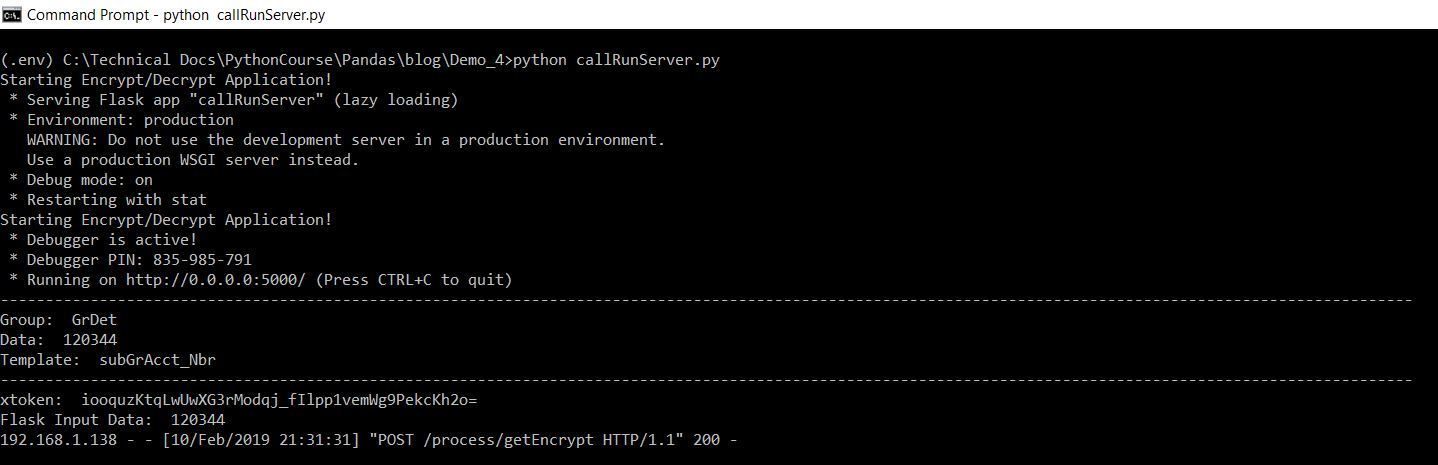And, we’re using Postman Third-party app to invoke this & please find the authentication details & JSON Payload for encrypting are as follows –Let’s see the decrypt from the server-side & how it looks like from the Postman –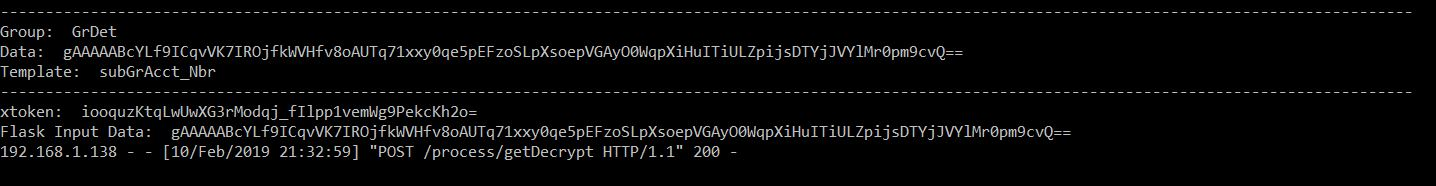Mac (32 bit):

Let’s look from MAC’s perspective & how the encryption debug looks like from the server.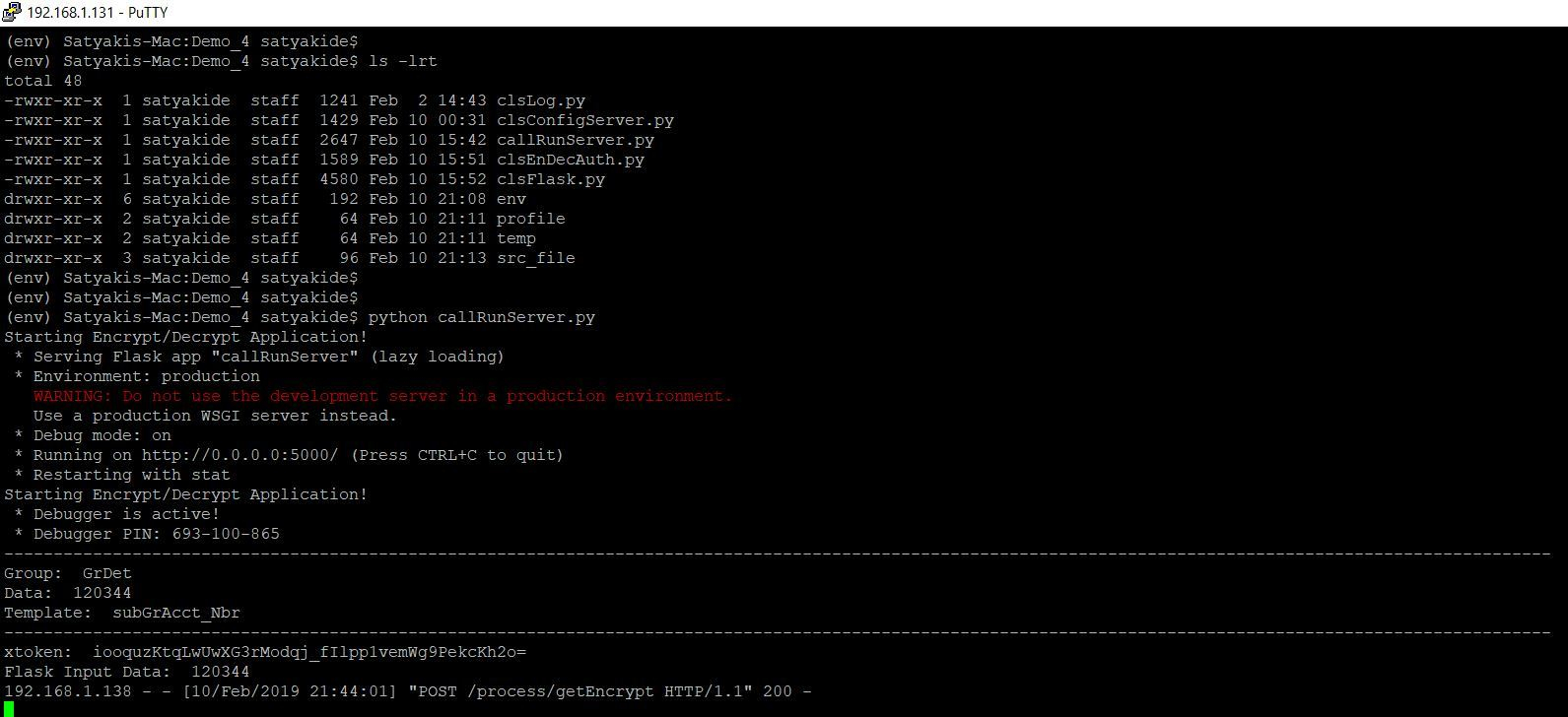Please find the screen from postman along with the necessary authentication –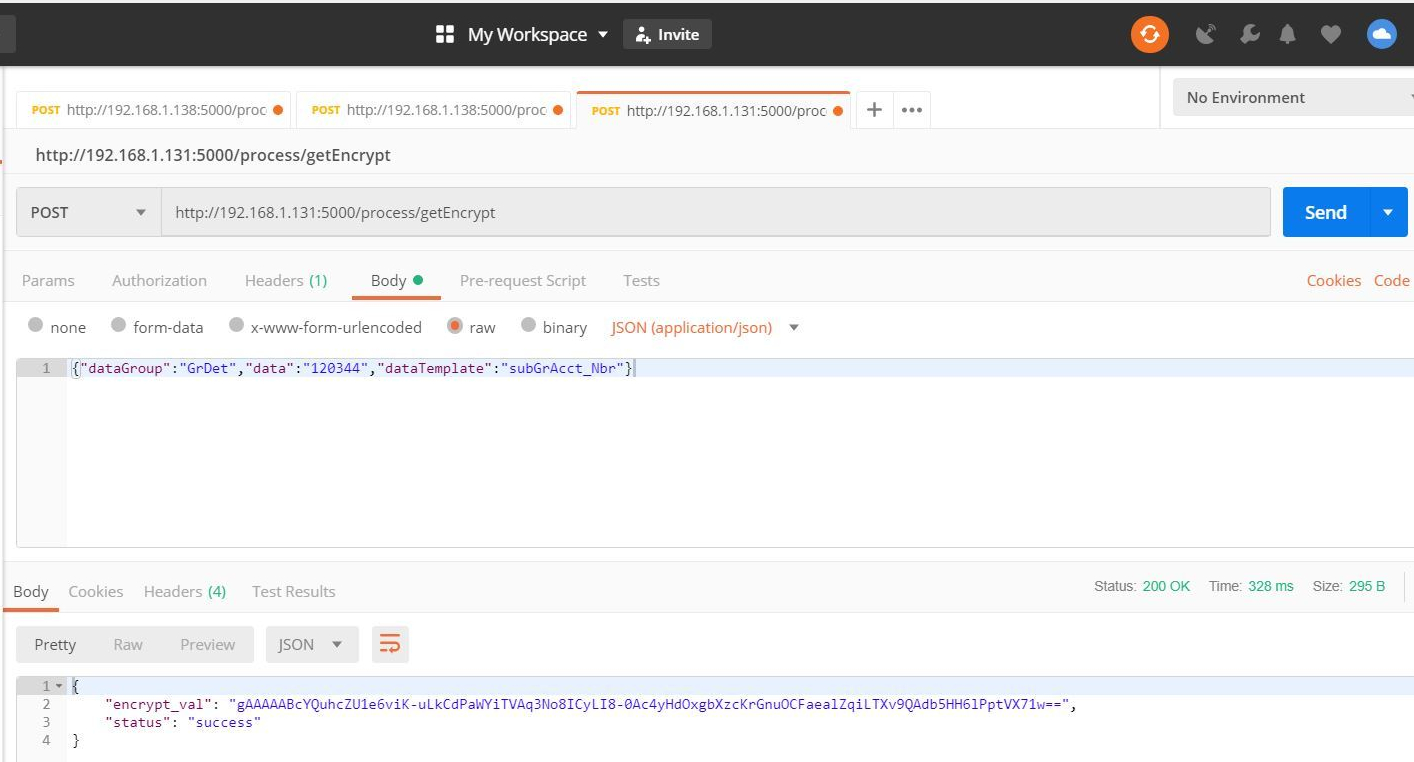Let’s discover how the decrypt looks like both from server & Postman as well –So, from this post, we’ve achieved our goal. We’ve successfully demonstrated of a creating a server component using Flask framework & we’ve incorporated our custom encryption/decryption script to create a simulated API for the third-party clients or any other application.

Hope, you will like this approach.

Let me know your comment on the same.

I’ll bring some more exciting topic in the coming days from the Python verse.

Till then, Happy Avenging!